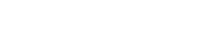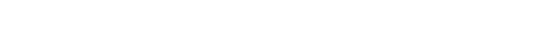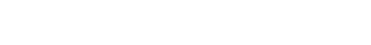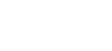1.1什幺是分类和回归

1.2两者区别与联系1、Logistic Regression 和 Linear Regression

Linear Regression：输出一个标量wx+b，是连续值，用以处理回归问题；

Logistic Regression：将标量wx+b通过sigmoid函数映射到(0,1)上，并划分一个阈值，大于阈值的分为一类，其他归为另一类，可处理二分分类问题；

2、Support Vector Regression 和 Support Vector Machine

SVR：输出wx+b，即某个样本点到分类面的距离，是连续值，用以处理回归问题；

SVM：将该距离通过sign(·)函数映射，距离为正的样本点为一类，为负的是另一类，故为分类问题。

1.3相应有哪些常用方法

（常见的聚类方法：K-Means(K均值)聚类等）

2.1逻辑回归

Logistic回归主要思想是，根据现有数据对决策边界建立回归方程，然后将回归方程映射到分类函数上实现分类。

2.2原理介绍

Logistic回归的原理可以理解为以下四步：

1、利用回归方程表示决策边界

，其中 W 代表权重向量。2、利用 Sigmoid 函数对回归关系进行映射

:3、在得到拟合函数后，利用损失函数来评价模型与实际值之间的差异大小4、求出损失函数取得极小值时对应的W ，从而得到拟合函数

2.3评价指标

1、混淆矩阵(confusion matrix)

positive)、假负FN(false negative)的数量，其中真正和假负均为正确分类的结果。2、准确率、真正率及假正率

ACC=1-ERR=TN+TP}/{TN+TP+FN+FP} 。

ROC曲线由变量1-Specificity和Sensitivity绘制，其中横轴1-Specificity=假正率(FPR)、纵轴Sensitivity=真正率(TPR)，ROC曲线的对角线表示随机猜测，若ROC曲线在对角线下表示分类器性能比随机猜测还差，ROC曲线下的区域面积(area
under the curve,AUC)表示分类模型的性能，反映了模型将正例排在反例前的比例（当AUC=1时，说明将所有正例均排在反例之前）。AUC同时考虑了学习器对于正例和负例的分类能力，在样本不平衡的情况下，依然能对分类器做出合理评价（如癌症预测）。3.1提出问题

3.2理解数据

1、导入包和数据

#1.导入包

import warnings

import pandas as pd

import numpy as np

from collections import OrderedDict

import matplotlib.pyplot as plt

warnings.filterwarnings(‘ignore’)

#2.创建数据（学习时间与是否通过考试）

‘考试成绩’:[0, 0, 0, 0, 0, 0, 0, 0, 0, 1, 0, 1, 1,
1, 1, 1, 1, 1, 1, 1]}

>>>

0  0.50  0

1  0.75  0

2  1.00  0

3  1.25  0

4  1.50  0

2、查看数据

#查看数据具体形式

#查看数据类型及缺失情况

>>>

<class ‘pandas.core.frame.DataFrame’>

RangeIndex: 20 entries, 0 to 19

Data columns (total 2 columns):

dtypes: float64(1), int64(1)

#查看描述性统计信息

>>>

count 20.00000 20.000000

mean 2.87500 0.500000

std 1.47902 0.512989

min 0.50000 0.000000

25% 1.68750 0.000000

50% 2.87500 0.500000

75% 4.06250 1.000000

max 5.25000 1.000000

3、绘制散点图查看数据分布情况

```#提取特征和标签
#绘制散点图
plt.scatter(exam_X,exam_y,color='b',label='考试数据')
plt.legend(loc=2)
plt.xlabel('学习时间')
plt.ylabel('考试成绩')
plt.show()```3.3构建模型

1、拆分训练集并利用散点图观察

#1.拆分训练集和测试集

from sklearn.cross_validation import train_test_split

exam_X =exam_X.values.reshape(-1,1)

exam_y =exam_y.values.reshape(-1,1)

train_X,test_X,train_y,test_y =train_test_split (exam_X,exam_y,train_size=0.8)

print (‘训练集数据大小为’, train_X.size,train_y.size)

print (‘测试集数据大小为’, test_X.size,test_y.size)

>>>

#2.散点图观察

plt.scatter (train_X,train_y, color=’b’, label=’train
data’)

plt.scatter (test_X,test_y, color=’r’, label=’test
data’)

#plt.plot (test_X,pred_y,color=’r’)

plt.legend(loc=2)

plt.xlabel(‘Hours’)

plt.ylabel(‘Scores’)

plt.show()2、导入模型

#3.导入模型

from sklearn.linear_model import LogisticRegression

modelLR=LogisticRegression()

3、训练模型

#4.训练模型

modelLR.fit(train_X,train_y)

3.4模型评估

1、模型评分（即准确率）

modelLR.score(test_X,test_y)

>>>

0.75

2、指定某个点的预测情况

#学习时间确定时，预测为0和1的概率分别为多少？

#学习时间确定时，预测为0和1的概率分别为多少？

modelLR.predict_proba(3)

>>>

array([[0.36720478, 0.63279522]])

#学习时间确定时，预测能否通过考试？

modelLR.predict(3)

>>>

array()

3、求出逻辑回归函数并绘制曲线

```#先求出回归函数y=a+bx，再代入逻辑函数中pred_y=1/(1+np.exp(-y))
b=modelLR.coef_
a=modelLR.intercept_
print('该模型对应的回归函数为:1/(1+exp-(%f+%f*x))'%(a,b))
>>>
该模型对应的回归函数为:1/(1+exp-(-1.527106+0.690444*x))```

```#画出相应的逻辑回归曲线
plt.scatter (train_X,train_y,color='b', label='train data')
plt.scatter (test_X,test_y,color='r', label='test data')
plt.plot (test_X,1/ (1+np.exp(-(a+b*test_X))),color='r')
plt.plot (exam_X,1/ (1+np.exp(-(a+b*exam_X))),color='y')
plt.legend(loc=2)
plt.xlabel('Hours')
plt.ylabel('Scores')
plt.show()```4、得到模型混淆矩阵

```from sklearn.metrics import confusion_matrix
#数值处理
pred_y=1/(1+np.exp(-(a+b*test_X)))
pred_y=pd.DataFrame(pred_y)
pred_y=round(pred_y,0).astype(int)
#混淆矩阵
confusion_matrix(test_y.astype(str),pred_y.astype(str))
>>>
array([[1, 1],
[0, 2]])```

5、绘制模型ROC曲线

from sklearn.metrics
import roc_curve, auc ###计算roc和auc

# Compute ROC curve and ROC area for each class

fpr,tpr,threshold = roc_curve(test_y, pred_y)
###计算真正率和假正率

roc_auc = auc(fpr,tpr) ###计算auc的值

plt.figure()

lw = 2

plt.figure(figsize=(10,10))

plt.plot(fpr, tpr, color=’r’,

lw=lw, label=’ROC curve (area = %0.2f)’ % roc_auc)
###假正率为横坐标，真正率为纵坐标做曲线

plt.plot([0, 1], [0, 1], color=’navy’, lw=lw,
linestyle=’–‘)

plt.xlim([0.0, 1.0])

plt.ylim([0.0, 1.0])

plt.xlabel (‘False Positive Rate’)

plt.ylabel (‘True Positive Rate’)

example’)

plt.legend (loc=”lower right”)

plt.show()